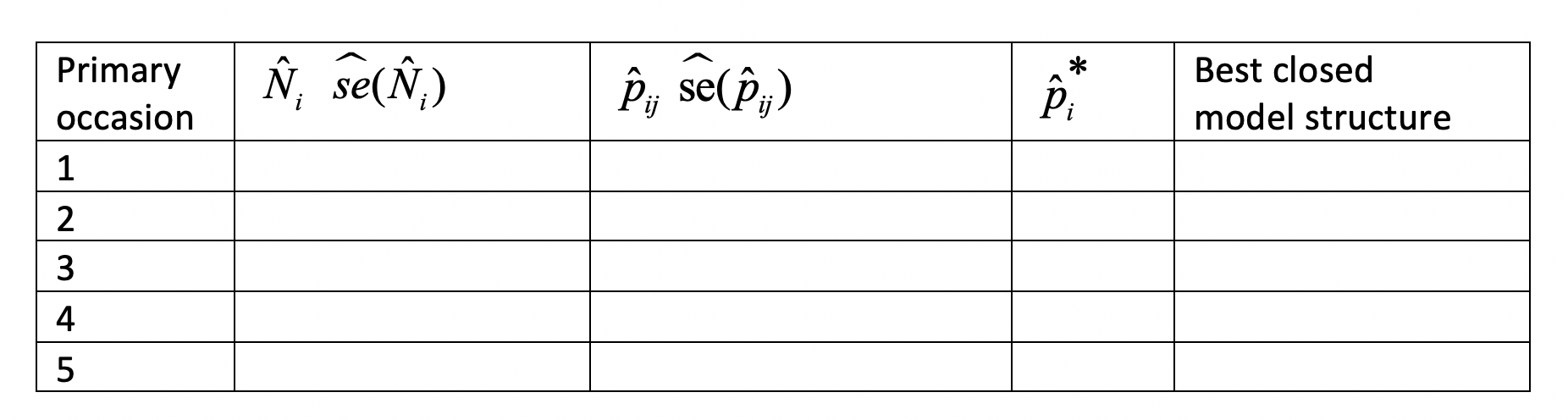For your homework for this week, please complete the assignment below by the beginning of class next Tuesday.

This week’s assignment is intended to introduce you to some of the core concepts of modeling with the robust design. We will use data from 5 primary occasions each of which has 3 secondary occasions. To help you see the connections between estimation based on the data from closed sessions and from open sessions, we will start with the ad-hoc approach. To do that we will (1) model the data from each of the closed sessions, (2) model the data from the primary sessions with CJS models, (3) examine the estimates of $$N_i$$, $$p_{i,j}$$, $$p_i^*$$ (from closed modeling), $$\phi_i$$, and $$p_i^{open}$$ (from CJS modeling), which in this homework we’ll call $$p_i^{CJS}$$ to try to avoid confusion, where $$i$$ represents the primary occasion and $$j$$ represents the secondary occasion within primary occasion $$i$$, (4) calculate temporary emigration rates from the estimates of $$p_i^*$$ and $$p_i^{CJS}$$. After doing that, we’ll model the same data using the robust-design model to see how the estimates to compare with those obtained from the ad-hoc approach and to see the benefits of using the likelihood approach.

The file “HW07.zip” contains information on live recaptures of adult females obtained during a study with 5 primary occasions that have 3 secondary occasions each. You will analyze the data in several ways: (1) using closed models for the data on animals captured during the secondary occasions within each of the primary occasions, (2) using live-recaptures models on data from the 5 primary occasions that collapses information across secondary occasions to a single 0 (never caught on any secondary occasion) or 1 (caught on at least one of the secondary occasions within a primary period), and (3) robust-design models.

1. Analyze the data from each of the primary occasions using closed-captures models with models M(0), M(t), and M(b). Record which model is best for each data set, and record the estimates of $$p_{i,j}$$ and $$N_i$$ for model M(0). Calculate $$p_i^*$$, where $$p_i^* = 1 - \displaystyle \prod_{j = 1}^{l_i}(1-p_{i,j})$$. Then, fill in the table below.1. Next, analyze the data in rd_simple1_cjs.inp with models that evaluate the presence of time variation in $$\phi$$, $$p$$, or both. Report which model is best supported and provide the estimates from the top model (we’ll ignore model-selection uncertainty for now).